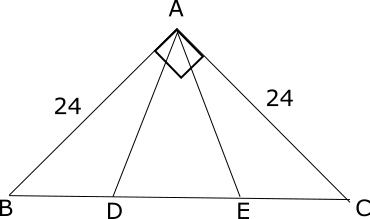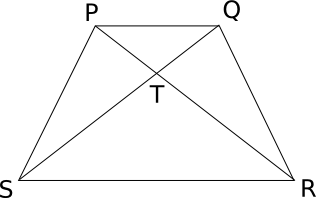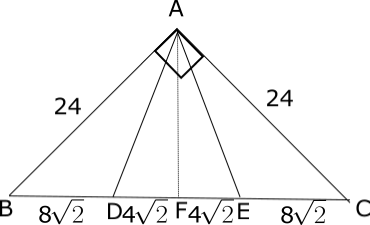# CAT Questions on Triangles – Geometry questions and answers

0
3542

## CAT Questions on Triangles:

Triangles questions and answers for CAT. Important Geometry questions based on Triangles from previous asked problems.

CAT Data Interpretation Questions PDF

Question 1: How many triangles with integral sides are possible with perimeter $$26 cm$$?

a) 13
b) 14
c) 18
d) 16

Question 2: How many scalene triangles exist with integral sides and sum of sides less than 13?

a) 3
b) 4
c) 5
d) None of these

Question 3: On a square ABCD, there are 3 points marked on the side AB (excluding A and B), 4 points marked on the side BC (excluding B and C), 5 points marked on the side CD (excluding C and D) and 2 points marked on the side DA ( excluding D and A). How many triangles can be formed from these points (including the vertices A, B, C and D)?

a) 487
b) 491
c) 747
d) 751

Question 4: In an isosceles trapezium PQRS, diagonals PR and QS intersect at T. The ratio of areas of triangles PQT and RST is 16 : 81. What is the ratio of the area of triangle PTS to the area of the trapezium PQRS?

a) 9 : 121
b) 18 : 121
c) 18 : 169
d) None of the above

Question 5: A right angled triangle with sides AB = AC = 24, is cut into three parts of equal area as shown in the figure given below. Find the sum of perimeters of all the triangles in the figure.a) 144 + 40$$\sqrt2$$ + 48$$\sqrt5$$
b) 144 + 80$$\sqrt2$$ + 48$$\sqrt5$$
c) 72 + 40$$\sqrt2$$ + 24$$\sqrt5$$
d) 72 + 40$$\sqrt2$$ + 48$$\sqrt5$$

Solutions for CAT Questions on Triangles:

Solutions:

Since the given perimeter is even, the number triangles possible with integral sides = $$[\frac{Perimeter^2}{48}]=[\frac{26\times26}{48}]=[14.08]=14$$
So the correct option to choose is B.

The concept to be used here is that the sum of any two sides of a triangle is greater than the third side.
When sum of sides is less than 13 the only possible triplets of sides are
(2,3,4), (2,4,5) and (3,4,5).

Total number of points on the square = 2 + 3 + 4 + 5 + 4 = 18 (including points A, B, C and D)
Of these 18 points, 5 points are collinear on side AB, 6 points are collinear on side BC, 7 points are collinear on side CD and 4 points are collinear on side DA.
So, the number of triangles that can be formed is $$^{18}C_3 – ^5C_3 – ^6C_3 – ^7C_3 – 4C_3$$ = 816 – 10 – 20 – 35 – 4 = 747
So, a total of 747 triangles can be formed from these points.In trapezium PQRS, area of triangle PQT : area of triangle RST = 16 : 81
=> PQ : RS = $$\sqrt{16 : 81}$$ = $$4 : 9$$
Let the two sides be 4k and 9k
Triangles PQT and RST are similar. Let the height of the trapezium (distance between the parallel sides) be 13h.
So, height of triangle PQT = 4t and height of triangle RST = 9t
Area of PQS = ½ * 4k * 13h
So, area of triangle PTS = ½ * 4k * 13h – ½ * 4k * 4h = 18kh
Area of the trapezium PQRS = ½ * sum of the parallel sides * distance between the parallel sides = ½ * (4k + 9k) * 13h = 169kh/2
So, required ratio = 18*2 : 169 = 36 : 169Since, $$\angle BAC=90^0, BC^2=AB^2+AC^2=576+576=1052$$
=> BC = 24$$\sqrt2$$
AF is the perpendicular as well as the median. Thus, BF = BD = 12$$\sqrt2$$.
Since, triangle AFB is perpendicular, $$AF^2 = AB^2 – BF^2 = 576 – 288$$
=> AF = $$12\sqrt{2}$$
Since, the area of the triangles ABD = ADE = AEC, BD = DE = EC = 24$$\sqrt2$$/3 = 8$$\sqrt2$$
Also, AF is the median of DE => DF = DE/2 = 8$$\sqrt2$$/2 = 4$$\sqrt2$$
Since, ADF is right angled, $$AD^2 = DF^2 + AF^2 = 32 + 288 = 320$$
=> AD = 8$$\sqrt5$$
The perimeter of the triangles = (48 + 24$$\sqrt2$$ ) + 2(24 + 8$$\sqrt2$$ + 8$$\sqrt5$$ ) + (8$$\sqrt2$$ + 2×8$$\sqrt5$$ ) + 2(24 + 16$$\sqrt2$$ + 8$$\sqrt5$$ ) = 144 + 80$$\sqrt2$$ + 48$$\sqrt5$$